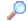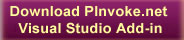SearchDirectory

Desktop Functions:

Smart Device Functions:GlossaryShow Recent ChangesSubscribe (RSS)Misc. Pages
MoveMemory (kernel32)

Summary
The MoveMemory function moves a block of memory from one location to another.

#### C# Signature:

[DllImport("Kernel32.dll", EntryPoint="RtlMoveMemory", SetLastError=false)]
static extern void MoveMemory(IntPtr dest, IntPtr src, int size);

#### VB Signature:

Declare Auto Sub MoveMemory Lib "Kernel32.dll" _
Alias "RtlMoveMemory" (ByVal dest As IntPtr, ByVal src As IntPtr, ByVal size As Integer)

None.

#### Alternative Managed API:

Use Marshal.Copy() twice to move from the unmanaged heap into a managed array and back out. Not ideal.

#### Sample Code:

<DllImport("kernel32.dll", EntryPoint:="RtlMoveMemory", SetLastError:=True, CharSet:=CharSet.Auto, ExactSpelling:=True, CallingConvention:=CallingConvention.StdCall)> _

Public Shared Sub CopyArrayTo(<[In](), MarshalAs(UnmanagedType.I4)> ByVal hpvDest As Int32, <[In](), Out()> ByVal hpvSource() As Byte, ByVal cbCopy As Integer)

End Sub

Public Function ConvertRBGTo1bpp(ByVal pSrcImg As Image) As Bitmap

Dim nColors As Integer = 2
Select Case nColors
Case nColors > 256
nColors = 256
Case nColors < 2
nColors = 2
End Select
Dim Width As Integer = pSrcImg.Width
Dim Height As Integer = pSrcImg.Height
Dim bitmap As Bitmap = New Bitmap(Width, Height, PixelFormat.Format1bppIndexed)
Dim BmpCopy As Bitmap = New Bitmap(Width, Height, PixelFormat.Format32bppArgb)
Dim g As Graphics
g = Graphics.FromImage(BmpCopy)
g.PageUnit = GraphicsUnit.Pixel
g.DrawImage(pSrcImg, 0, 0, Width, Height)
g.Dispose()
Dim bitmapData As BitmapData
Dim rect As Rectangle = New Rectangle(0, 0, Width, Height)
bitmapData = bitmap.LockBits(rect, ImageLockMode.WriteOnly, PixelFormat.Format1bppIndexed)
Dim pixels As IntPtr = bitmapData.Scan0
Dim bits As Byte()
Dim pBits As Int32
If (bitmapData.Stride > 0) Then
pBits = pixels.ToInt32()
Else
pBits = pixels.ToInt32() + bitmapData.Stride * (Height - 1)
End If
Dim stride As Integer = Math.Abs(bitmapData.Stride)
ReDim bits(Height * stride) ' Allocate the working buffer.
Dim row As Integer
Dim col As Integer
For row = 0 To Height - 1
For col = 0 To Width - 1
Dim pixel As Color ' The source pixel.
Dim i8BppPixel As Integer = (row * stride) + Int(col / 8)
bmask = &H80 / (2 ^ (col Mod 8))
pixel = BmpCopy.GetPixel(col, row)
Dim luminance As Double = (pixel.R * 0.299) + (pixel.G * 0.587) + (pixel.B * 0.114)
Dim colorIndex As Double = Math.Round((luminance * (nColors - 1) / 255))
If colorIndex = 0 Then
bits(i8BppPixel) = bits(i8BppPixel) Or (bmask And 0) 'Black
Else
bits(i8BppPixel) = bits(i8BppPixel) Or (bmask Or 0) 'White
End If
Next col
Next row
CopyArrayTo(pBits, bits, Height * stride)
bitmap.UnlockBits(bitmapData)
BmpCopy.Dispose()
Return bitmap

End Function

Documentation
MoveMemory on MSDN

Do you have...

• helpful tips or sample code to share for using this API in managed code?
• corrections to the existing content?
• variations of the signature you want to share?
• additional languages you want to include?

Select "Edit This Page" on the right hand toolbar and edit it! Or add new pages containing supporting types needed for this API (structures, delegates, and more).Access PInvoke.net directly from VS:Terms of UseEdit This PageFind ReferencesShow Printable VersionRevisions Show changes